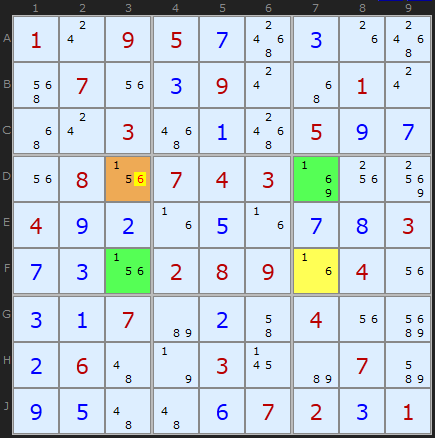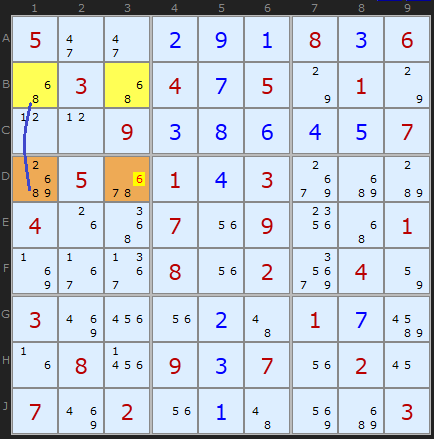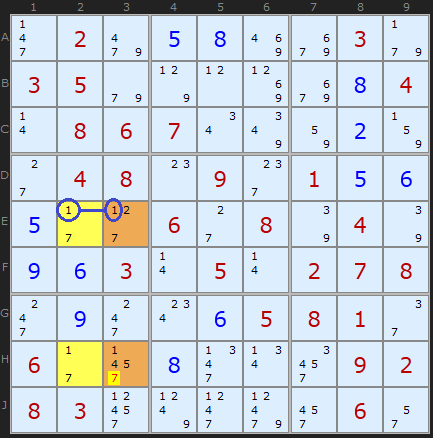Main Page - Back

# Hidden Unique Rectangles

From sudokuwiki.org, the puzzle solver's siteAll the Unique Rectangles discussed on this page have had at least two bi-value cells (or a Naked Pair, if you like). An interesting situation emerges when we have only one bi-value cell and the Unique Rectangle is buried, or 'hidden', under lots of other candidates. The conditions have to be just right, but this formation is surprisingly common.Hidden Unique Rectangle Type 1 : Load Example or : From the Start In Figure 1, the cells D3, D7, F3 and F7 (also known as DF37) form a rectangle with a potential deadly pattern on [1/6]. We want to avoid reducing this rectangle to 1/6 in all four cells. There is a bi-value cell at F7, but the other corners contain a clutter of other candidates.

The types of Unique Rectangles that we've examined so far simply won't work here. However, all is not lost because something interesting is going on in the corner opposite to F7. 1 in D3 is part of two strong links in the row and column - that is, 1 occurs only twice in row D and column 3. Our 1 in this rectangle can have only one of two possibilities.

Looking at D3, lets make "Option A" the case where D3 is 1. The pairs or "strong links" remove 1 from in D7 and F3. Option B is where D3 is not a 1. That would put a 1 in D7 and F3.
Let us conjecture that the solution to D3 is 6. That forces the 1s to take on option B because they would be the only remaining 1s in that row and column (D7 and F3). The implication of this option is that the only candidate for F7 is 6. But, wait a moment - this is exactly the deadly pattern we need to avoid - two numbers in two rows, two columns and two boxes. Since none of these cells is a clue, the puzzle could have two solutions. We can swap the 1 and the 6 around. This makes option B unviable.

So surely we can fix the 1s in D7 and F3? Not quite. We haven't taken into account the clutter of other clues in the corners of this rectangle. But we can say something about the 6 in D3. We've excluded it for the reason above, but option A also excludes the 6 in D3. Whichever way round F7 is, D3 cannot contain a 6, so we can remove it from that cell.

## Type 2 Hidden Unique RectanglesFigure 2, Hidden Unique Rectangle Type 2 : Load Example or : From the Start In Figure 2, the cells B1, B3, D1 and D3 (also known as BD13) form a rectangle with a potential deadly pattern on [6/8]. In type 2 we have an identifiable Floor consisting of B1 and B3, both bi-value cells. And the Roof contains a clutter of other candidates. We still want to avoid reducing this rectangle to 6/8 in all four cells.

Type 2 starts with a Naked Pair, 8/6, and we're checking for which strong links exist on the pair candidates. The Naked Pair is obviously a double strong link on both numbers, but candidate 8 has a strong link to the Roof in column 1. From this we can start using the logic of Type 1.

Is 6 viable for either Roof Cells? D1 is viable since D3 does not have to be a 8. However, D3 as 6 is trouble because it forces B3 to be 8, B1 to be 6 and because of the strong link, D1 becomes 8. A Deadly Pattern, so it is safe to remove 6 from D3. At first glance the formation looks symmetrical but its the other 6s and 8s (or lack of) in the columns that give us the implications of the Hidden Rectangle.

## Type 2b Hidden Unique RectanglesHidden Unique Rectangle 2b : Load Example or : From the Start In common with Unique Rectangles there is a type B for this strategy, where the floor is across two boxes rather than in the same box.

The yellow cells are the floor with conjugate pairs 1/7. The Deadly Rectangle extends to E3 and H3 (orange) where 1 and 7 are also present among other candidates. The strong link on 1 between E2 and E3 means that a 7 in H3 would create a Deadly Rectangle.

Thanks to Jerry Foil of Virginia, USA for providing the first example. Interestingly this type of Hidden Unique Rectangle is almost twice as common as the Type 1 and three times as common as Type 2.